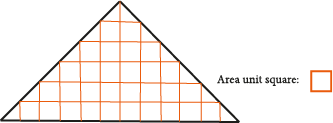## Posts

Showing posts from December, 2018

### Expansion of FractionsExpansion of Fractions The process of making a unit fraction smaller is called expansion. You may say, ‘How is it that the process of fraction becoming smaller is called expansion, isn’t this strange?’ Let’s find out; Let the fraction be;  $$\displaystyle \frac{2}{3}$$ The unit fraction of   $$\displaystyle \frac{2}{3}$$  is  $$\displaystyle \frac{1}{3}$$ Let’s expand this fraction by 2 without changing its value. We will carry out the expansion with multiplication. We know this; when we multiply a unit/ a number by 1, it remains the same, it doesn’t change. So, in fact, we do not multiply with 2 but we multiply with 1.

### Why is the total value of the angles of triangles 180°?Why is the total value of the angles of triangles 180°? The total value of internal angles of all triangles, no matter what type, equals to 180°; let’s try and prove why this is the case. Before the proof, we must remember the angles of two parallel lines at intercepts. The angles that are formed when two parallel lines intersect are equal to each other. The intersecting line continues to cut the lines and comes towards the other parallel line. Let’s create a similar system for a triangle; Let’s create a triangle, showing the angles in different colors, and draw a line parallel to the base. Now let us look at the situation of the angles and carry out the ‘moving’ operation. Let’s focus at the very top, the blue, yellow and red angles show the total value of the angles. Since the horizontal line has an angle of 180°, the total value of the angles in a triangle is equal to 180°

### How to read fractions ?$$\displaystyle \frac{1}{2}$$ "one half" or "a half" $$\displaystyle \frac{1}{3}$$ "one third" or "a third" $$\displaystyle \frac{1}{4}$$ "one quarter" or "a quarter" $$\displaystyle \frac{2}{3}$$ "two third s " not "two third" ( 2 is plural so we add "s" ) . $$\displaystyle \frac{2}{5}$$  "two fifth s " $$\displaystyle \frac{3}{7}$$ " three sevenths" $$\displaystyle \frac{5}{6}$$ " five sixths" $$\displaystyle \frac{3}{4}$$ " three quarters" $$\displaystyle \frac{11}{10}$$  "eleven tenths" $$\displaystyle 2\frac{9}{10}$$ " two and nine tenths"  is like $$\displaystyle \text 2 whole$$ and  $$\displaystyle \text nine$$ $$\displaystyle \frac{1}{10}$$

### ExponentsEarlier you had learnt that adding the same number over and over again is the same thing as multiplication. For example; $$\displaystyle 4+4+4+4+4+4+4$$ instead of this equation we can use multiplication.  Because we are adding the number 4, 7 times:   $$\displaystyle 4+4+4+4+4+4+4=7.4$$ Now, we are going to focus on multiplying the same number several times. We are going to develop methods to make this easy for us as well as to show this in a simple way.

### The area of triangleWe measure an area with squares; similarly we calculate the area of a triangle in the same way. The area of a triangle is calculated by seeing how many squares can fit inside the triangle. The triangle above consists of 30 unit squares and 12 half squares. Since 12 half squares equal 6 whole squares, we can say that the triangle above is 36 unit squares.

### Ordering and comparing rational numbersTo order / compare rational numbers; a )You can order rational numbers either logically, based on  its division. b) You can think of a rational number as a fraction and then sort it. The way we sort fractions is the same way to sort rational numbers. We recommend that you also look at the topic of ordering in fractions.